Topics: AI | Data Science | Data Visualization | Deep Learning | Machine Learning | NLP | Python | R | Statistics

KDnuggets Home » News » 2017 » Oct » Tutorials, Overviews » A Guide For Time Series Prediction Using Recurrent Neural Networks (LSTMs) ( 17:n39 )

# A Guide For Time Series Prediction Using Recurrent Neural Networks (LSTMs)

http likes 137

Looking at the strengths of a neural network, especially a recurrent neural network, I came up with the idea of predicting the exchange rate between the USD and the INR.

By Neelabh Pant, Statsbot.

Note: The Statsbot team has already published the article about using time series analysis for anomaly detection. Today, we’d like to discuss time series prediction with a long short-term memory model (LSTMs). We asked a data scientist, Neelabh Pant, to tell you about his experience of forecasting exchange rates using recurrent neural networks.As an Indian guy living in the US, I have a constant flow of money from home to me and vice versa. If the USD is stronger in the market, then the Indian rupee (INR) goes down, hence, a person from India buys a dollar for more rupees. If the dollar is weaker, you spend less rupees to buy the same dollar.

If one can predict how much a dollar will cost tomorrow, then this can guide one’s decision making and can be very important in minimizing risks and maximizing returns. Looking at the strengths of a neural network, especially a recurrent neural network, I came up with the idea of predicting the exchange rate between the USD and the INR.
There are a lot of methods of forecasting exchange rates such as:

• Purchasing Power Parity (PPP), which takes the inflation into account and calculates inflation differential.
• Relative Economic Strength Approach, which considers the economic growth of countries to predict the direction of exchange rates.
• Econometric model is another common technique used to forecast the exchange rates which is customizable according to the factors or attributes the forecaster thinks are important. There could be features like interest rate differential between two different countries, GDP growth rates, income growth rates, etc.
• Time series model is purely dependent on the idea that past behavior and price patterns can be used to predict future price behavior.

In this article, we’ll tell you how to predict the future exchange rate behavior using time series analysis and by making use of machine learning with time series.

### Sequence problems

Let us begin by talking about sequence problems. The simplest machine learning problem involving a sequence is a one to one problem.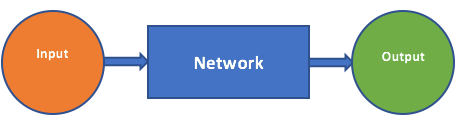One to One

In this case, we have one data input or tensor to the model and the model generates a prediction with the given input. Linear regression, classification, and even image classification with convolutional network fall into this category. We can extend this formulation to allow for the model to make use of the pass values of the input and the output.

It is known as the one to many problem. The one to many problem starts like the one to one problem where we have an input to the model and the model generates one output. However, the output of the model is now fed back to the model as a new input. The model now can generate a new output and we can continue like this indefinitely. You can now see why these are known as recurrent neural networks.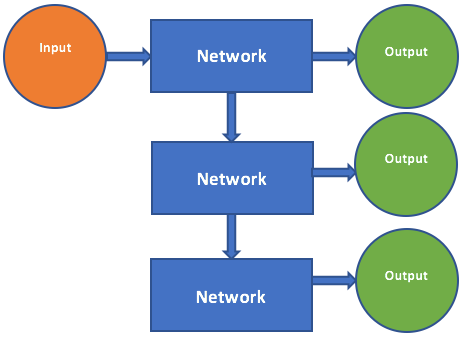One to Many

A recurrent neural network deals with sequence problems because their connections form a directed cycle. In other words, they can retain state from one iteration to the next by using their own output as input for the next step. In programming terms this is like running a fixed program with certain inputs and some internal variables. The simplest recurrent neural network can be viewed as a fully connected neural network if we unroll the time axes.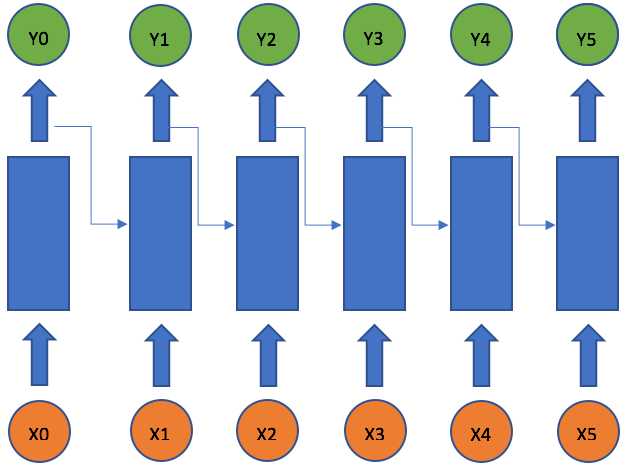RNN Unrolled Time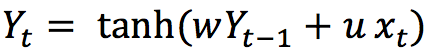In this univariate case only two weights are involved. The weight multiplying the current input xt, which is u, and the weight multiplying the previous output yt-1, which is w. This formula is like the exponential weighted moving average (EWMA) by making its pass values of the output with the current values of the input.

One can build a deep recurrent neural network by simply stacking units to one another. A simple recurrent neural network works well only for a short-term memory. We will see that it suffers from a fundamental problem if we have a longer time dependency.

### Long Short-Term Neural Network

As we have talked about, a simple recurrent network suffers from a fundamental problem of not being able to capture long-term dependencies in a sequence. This is a problem because we want our RNNs to analyze text and answer questions, which involves keeping track of long sequences of words.

In late ’90s, LSTM was proposed by Sepp Hochreiter and Jurgen Schmidhuber, which is relatively insensitive to gap length over alternatives RNNs, hidden markov models, and other sequence learning methods in numerous applications.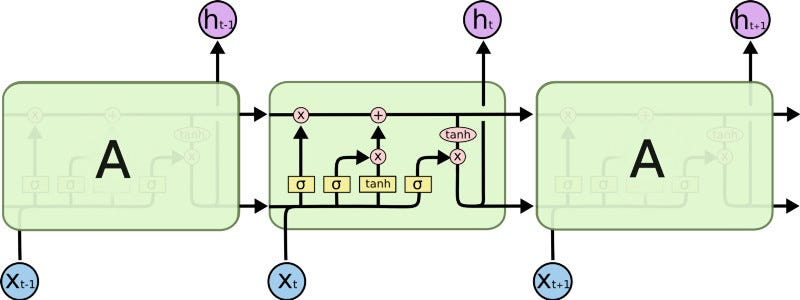LSTM Architecture (Source)

This model is organized in cells which include several operations. LSTM has an internal state variable, which is passed from one cell to another and modified by Operation Gates.

1. Forget Gate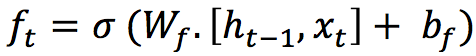It is a sigmoid layer that takes the output at t-1 and the current input at time tand concatenates them into a single tensor and applies a linear transformation followed by a sigmoid. Because of the sigmoid, the output of this gate is between 0 and 1. This number is multiplied with the internal state and that is why the gate is called a forget gate. If ft=0 then the previous internal state is completely forgotten, while if ft=1 it will be passed through unaltered.

2. Input Gate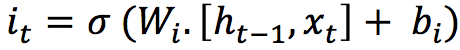The input gate takes the previous output and the new input and passes them through another sigmoid layer. This gate returns a value between 0 and 1. The value of the input gate is multiplied with the output of the candidate layer.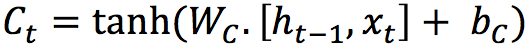This layer applies a hyperbolic tangent to the mix of input and previous output, returning a candidate vector to be added to the internal state.

The internal state is updated with this rule: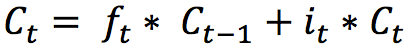The previous state is multiplied by the forget gate and then added to the fraction of the new candidate allowed by the output gate.

3. Output Gate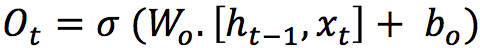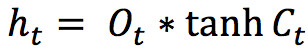This gate controls how much of the internal state is passed to the output and it works in a similar way to the other gates.

These three gates described above have independent weights and biases, hence the network will learn how much of the past output to keep, how much of the current input to keep, and how much of the internal state to send out to the output.

In a recurrent neural network, you not only give the network the data, but also the state of the network one moment before. For example, if I say “Hey! Something crazy happened to me when I was driving” there is a part of your brain that is flipping a switch that’s saying “Oh, this is a story Neelabh is telling me. It is a story where the main character is Neelabh and something happened on the road.” Now, you carry a little part of that one sentence I just told you. As you listen to all my other sentences you have to keep a bit of information from all past sentences around in order to understand the entire story.

Another example is video processing, where you would again need a recurrent neural network. What happens in the current frame is heavily dependent upon what was in the last frame of the movie most of the time. Over a period of time, a recurrent neural network tries to learn what to keep and how much to keep from the past, and how much information to keep from the present state, which makes it so powerful as compared to a simple feed forward neural network.

Top Stories Past 30 Days
Most SharedGet KDnuggets, a leading newsletter on AI, Data Science, and Machine Learning